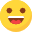# 算法日常：两数之和

``````function twoSum(\$num, \$target) {
for (\$i = 0; \$i < count(\$num); \$i++) {
for (\$j = 1; \$j < count(\$num); \$j++) {
if (\$num[\$i] + \$num[\$j] == \$target) {
\$res = \$i;
\$res = \$j;
//加个判断是为了避免同一元素二次利用，当然也是为了通关,,,,
if (\$i != \$j) {
return \$res;
}

}
}
}
return [];
}``````

#### 文章评论

* 必填